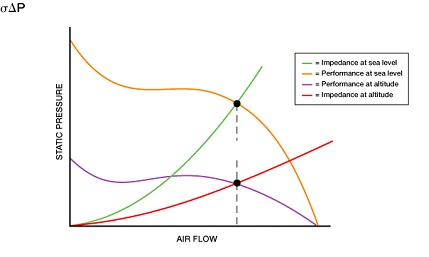Skip to content

# Altitude Operation

• Cooling Effect is directly proportional to air density,
• I.e. number of air molecules available.
• Air flow must be increased at altitude to provide the necessary molecules, in direct proportion to loss of density.
The selection process:
• Determine required air flow at the altitude density.
• Determine your system impedance for this air flow at sea level conditions.
• Select air mover from sea level test data using 1. & 2. Above
• Evaluate the density range of operation, as it may be possible to reduce motor size, due to decreased load at altitude.
Under constant speed conditions:
• Air flow is constant between sea level and any altitude.
• Static Pressure is also directly proportional to air density.
• Static Pressure at altitude will be less than at sea level, but flow remains constant because system resistance is decreased by the same density ratio.
• Power consumption at altitude is reduced in direct proportion to density, due to the direct reduction of static pressure.
• Selecting an air mover to meet the altitude cooling needs, always results in excess performance at sea level.
• Excess weight
• Excess power consumption
• Excess acoustic noise
• Excess wear and tear, reduced life
• Consider a variable speed or Altivar fan to reduce speed at lower altitudes.
• Variable speed fans utilize electronic drive and control.Density Calculation:

r = .75 (Pb / 29.92) X (530 / t)
Where r = density in lb. per cubic foot
t = Rankine temperature of (°F + 460)
Pb = barometric pressure in inches of Hg

For sea level to 29,000 foot altitude:
Pb = 29.873 – 1.049A ­+ .012A2 3% max. error
Where A = altitude in thousands of feet

For 30,000 to 60,000 foot altitude:
Pb = 24.021 – 1.049A + .012A2 3% max. error